# Determination of Optimal Gelling Agent Concentration

Gels are widely-used in formulations because their unique features help in enabling stability and creating textures in products. Biopolymers are oftentimes used for this gel formation process. In optimally using gels in various projects, the following questions should be asked first:

1. What is the minimum gelling agent concentration required in the formulation of a gel?
2. What is the gel point for a given concentration?
3. What is the gel strength of each gel?
4. How much time should be devoted in order to match a gel with a given reference elasticity level?

In order to answer these questions, a formulator needs to study many samples. This article presents with a sample of 12 different concentrations to be analyzed. The study of such number of samples using conventional analytical tools—such as mechanical rheology—is usually time-consuming; however, the Rheolaser MASTER is able to reduce processing time by analyzing 6 samples simultaneously. In just one click, the gel point, its minimum concentration, and the elasticity of the samples could be determined.

Rheolaser Master - Formulaction - Explore rheology at the nano scale from Formulaction on Vimeo.

Presented herein is a study on a simple liquid system of liquid phase and a gelling agent (milk with glucono-δ-lactone/GDL) that may be generalized for any type of gelling system.

## What is the Minimum Concentration of a Gelling Agent to Obtain a Gel?

Rheolaser measures the particle Mean Square Displacement (MSD), a method that provides details on the viscoelastic properties of the material. The data on the MSD of various gelling agent concentrations are presented below. The curves in the top left signify gelling agents with low concentration. Their linear shape indicates a purely liquid behavior. Meanwhile, an increase in concentration formulates new gels, leading to a typical viscoelastic mean square displacement called plateau formation.

The minimal concentration of the gelling agent is determined using the Time-Cure Superposition (TCS) method which is done by rescaling the MSD curves, thereby yielding characteristic v-shaped curves that would indicate the minimum gelling agent concentration.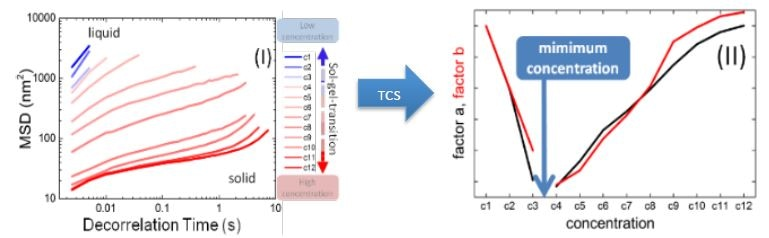Figure 1. (I) MSD curves of different gelling agent concentrations. (II) TCS results giving concentration to create a gel.

## What i the Gel Point for the Given Concentration?

As previously reported, samples with concentrations C4 to C12 were found to form a gel, with the gelling time for each concentration determined using the TCS method. Various gel points are listed in the formulation matrix found in the latter part of this article.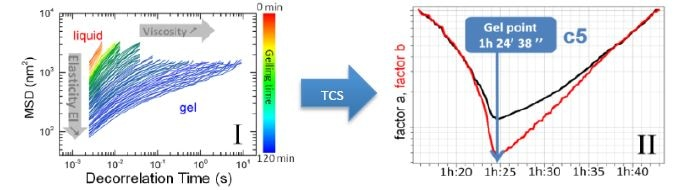Figure 2. (I) Evolution of MSD curves during gelation. (II) TCS results giving time necessary to create a gel.

## What is the Elastic Strength of Each Level? How Long Would it Take to Reach this Level?

Rheolaser also measure viscoeslatic properties such as the elasticity index (EI). In this case, a higher EI would result to a higher gel elasticity. The figure presented shows the EI evolution of various concentrations and the gel strength of each gel is listed in the formulation matrix below. Rheolaser is also able to compare various samples kinetically and to determine the specific time to reach 90% of a given reference elasticity. In the presented example, C12 reaches 80% of the reference elasticity after 2 hours 8 mins; meanwhile, C8 reaches it after 4 hours and 46 mins because it is less concentrated.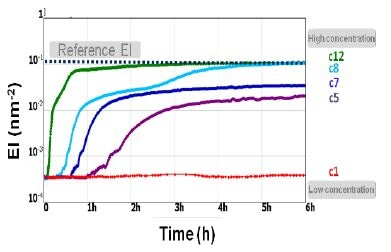## Creating a Formulation Matrix

A formulation matrix presents all previous results of a study. Through this device, the formulator could decide on the optimal composition of the product, depending on the formulation strategy. In the studied sample, C8 was found to be a relatively less-expensive formulation and is product-saving, whereas C12 is time-saving and yields a relatively faster production cycle. The 12 concentrations studied were measured in two batches of 6 samples each. An operator took less than 10 minutes to launch the measurements and interpret results. This is a much better process compared to a typical 2-hour time period with conventional instruments.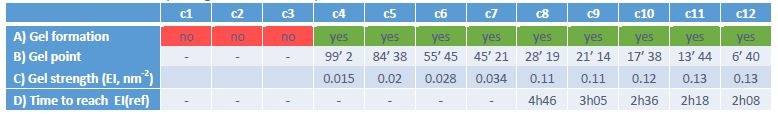## Possible Applications

Any industry that uses gelling systems, including:

• Food
• Pharmaceuticals
• Home
• Personal care

## Benefits

• Fast – able to measure up to 6 gels at a time, automatic data treatment
• Accurate – provides an exact measure of the gel point
• Easy - disposable measurement cells, closed environment, 1-click computation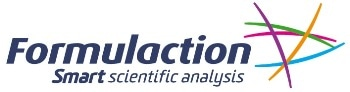This information has been sourced, reviewed and adapted from materials provided by Formulaction.

## Citations

• APA

Formulaction. (2019, October 15). Determination of Optimal Gelling Agent Concentration. AZoM. Retrieved on July 09, 2020 from https://www.azom.com/article.aspx?ArticleID=16247.

• MLA

Formulaction. "Determination of Optimal Gelling Agent Concentration". AZoM. 09 July 2020. <https://www.azom.com/article.aspx?ArticleID=16247>.

• Chicago

Formulaction. "Determination of Optimal Gelling Agent Concentration". AZoM. https://www.azom.com/article.aspx?ArticleID=16247. (accessed July 09, 2020).

• Harvard

Formulaction. 2019. Determination of Optimal Gelling Agent Concentration. AZoM, viewed 09 July 2020, https://www.azom.com/article.aspx?ArticleID=16247.

## Tell Us What You Think